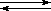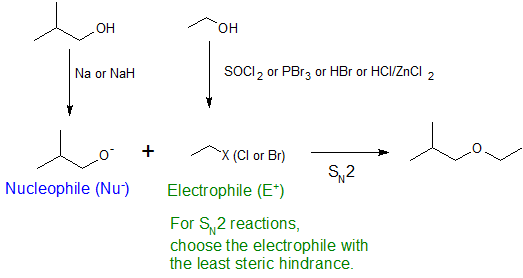# 10.10: Reactions of Alkoxides

$$\newcommand{\vecs}{\overset { \rightharpoonup} {\mathbf{#1}} }$$ $$\newcommand{\vecd}{\overset{-\!-\!\rightharpoonup}{\vphantom{a}\smash {#1}}}$$$$\newcommand{\id}{\mathrm{id}}$$ $$\newcommand{\Span}{\mathrm{span}}$$ $$\newcommand{\kernel}{\mathrm{null}\,}$$ $$\newcommand{\range}{\mathrm{range}\,}$$ $$\newcommand{\RealPart}{\mathrm{Re}}$$ $$\newcommand{\ImaginaryPart}{\mathrm{Im}}$$ $$\newcommand{\Argument}{\mathrm{Arg}}$$ $$\newcommand{\norm}{\| #1 \|}$$ $$\newcommand{\inner}{\langle #1, #2 \rangle}$$ $$\newcommand{\Span}{\mathrm{span}}$$ $$\newcommand{\id}{\mathrm{id}}$$ $$\newcommand{\Span}{\mathrm{span}}$$ $$\newcommand{\kernel}{\mathrm{null}\,}$$ $$\newcommand{\range}{\mathrm{range}\,}$$ $$\newcommand{\RealPart}{\mathrm{Re}}$$ $$\newcommand{\ImaginaryPart}{\mathrm{Im}}$$ $$\newcommand{\Argument}{\mathrm{Arg}}$$ $$\newcommand{\norm}{\| #1 \|}$$ $$\newcommand{\inner}{\langle #1, #2 \rangle}$$ $$\newcommand{\Span}{\mathrm{span}}$$$$\newcommand{\AA}{\unicode[.8,0]{x212B}}$$

## Introduction

The hydrogen atom of a hydroxyl group is ionizable and can be replaced by other substituents as illustrated in the reactions below. The first reaction shows simple alcohols with sodium (and sodium hydride). The second reaction shows the isotopic exchange that occurs when mixing an alcohol with deuterium oxide (heavy water). This exchange, which is catalyzed by acid or base, is rapid under normal conditions because it is difficult to avoid traces of these catalysts in most experimental systems.

 2 R–O–H + 2 Na2 R–O(–)Na(+) + H2 R–O–H + D2OR–O–D + D–O–H

The mechanism by which these substitution reactions proceed is straightforward. The oxygen atom of an alcohol is nucleophilic; therefore, it is prone to react with electrophiles. The resulting "onium" intermediate then loses a proton to a base, forming the substitution product. If a strong electrophile is not present, then the nucleophilicity of the oxygen may be enhanced by conversion to its conjugate base (an alkoxide). This powerful nucleophile then reacts with weak electrophiles. These two variations of the substitution mechanism are illustrated in the following diagram.## Williamson Ether Synthesis

Alkyl substitution of the hydroxyl group creates ethers. This reaction provides examples of both strong electrophilic substitution (first equation below) and weak electrophilic substitution (second equation). The latter SN2 reaction is known as the Williamson ether synthesis and is generally only used with 1º alkyl halide reactants because the strong alkoxide base leads to E2 elimination with 2º and 3º alkyl halides.Exercise

16. Show how you would use the Williamson Ether synthesis to make 1-ethoxy-2-methylpropane from isobutyl alcohol and ethanol.